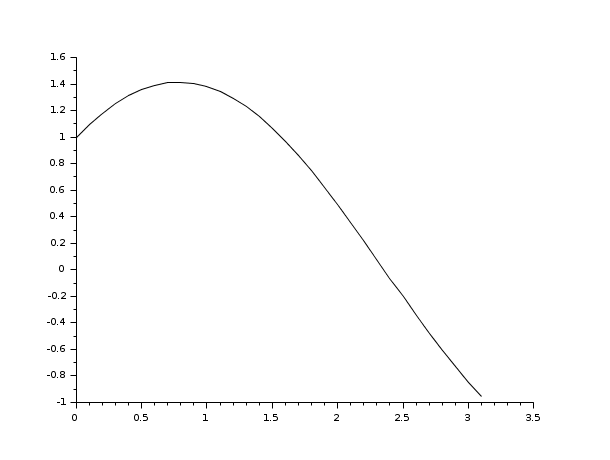Change language to:
English - Français - 日本語 - Português -

Справка Scilab >> Графики > 2d_plot > fplot2d

# fplot2d

2D plot of a curve defined by a function

### Calling Sequence

```fplot2d(xr,f,[style,strf,leg,rect,nax])
fplot2d(xr,f,<opt_args>)```

### Arguments

xr

vector.

f

external of type `y=f(x)` i.e. a scilab function or a dynamically linked routine referred to as a string.

style,strf,leg,rect,nax

see `plot2d`

<opt_args>

see `plot2d`

### Description

`fplot2d` plots a curve defined by the external function `f`. The curve is approximated by a piecewise linear interpolation using the points `(xr(i),f(xr(i))`. The values of f(x) are obtained by `feval(xr,f)`.

Enter the command `fplot2d()` to see a demo.

### Sample### Examples

```deff("[y]=f(x)","y=sin(x)+cos(x)")
x=[0:0.1:10]*%pi/10;
fplot2d(x,f)``````clf();
deff("[y]=f(x)","y=sin(x)+cos(x)")
x=[0:0.1:10]*%pi/10;
fplot2d(x,f)
fplot2d(1:10,'parab')```